# Make discoveries with your data

### Recent posts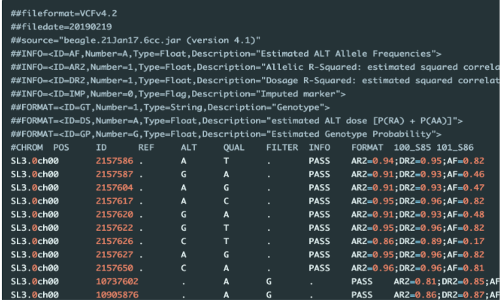## Annotation of genetic variants (SNPs/InDels) in VCF file

Genetic variant annotation for variant location in the genome, associated genes, and their gene functions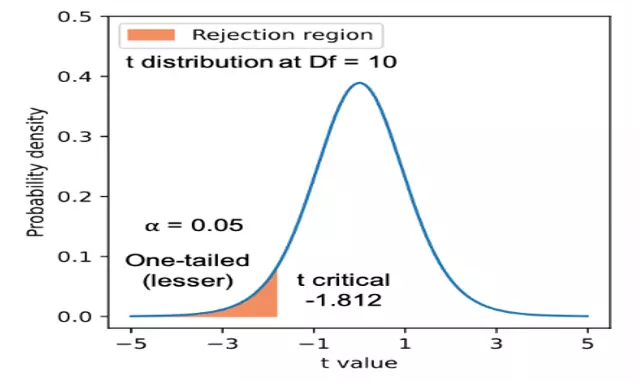## Statistical hypothesis testing, types of errors, and interpretation of p values

Learn how to propose null and alternate hypotheses, perform the statistical analysis, and interpret the results

## Query pandas DataFrame to select rows based on value and condition matching

Learn how to query pandas DataFrame to select rows based on exact match, partial match, and conditional match in pandas DataFrame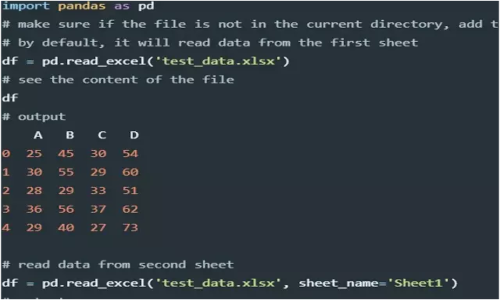## Import small and large data using pandas (CSV, Excel, Tab, JSON, SQL, and Parquet files)

learn how to import CSV, Excel, Tab, JSON, and SQL files in pandas for data analysis and visualization## Checking and handling missing values (`NaN`) in pandas

Analyse and handle null or missing values in pandas series and dataframe## Left, right, outer and inner joins on pandas dataframes

learn to join pandas dataframes in multiple ways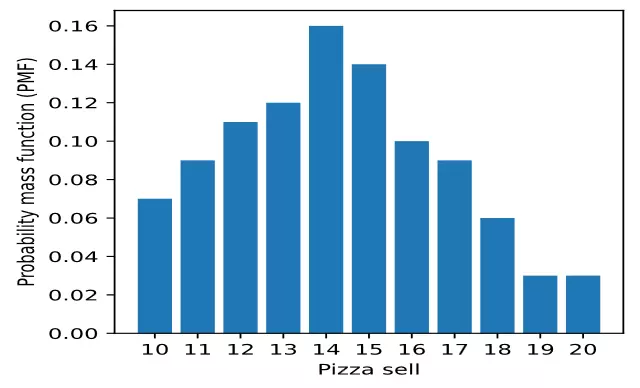## Find probabilities using discrete and continuous probability distributions

Learn how to use probability distributions, probability mass function (PMF), cumulative distribution function (CDF), and probability density function (PDF)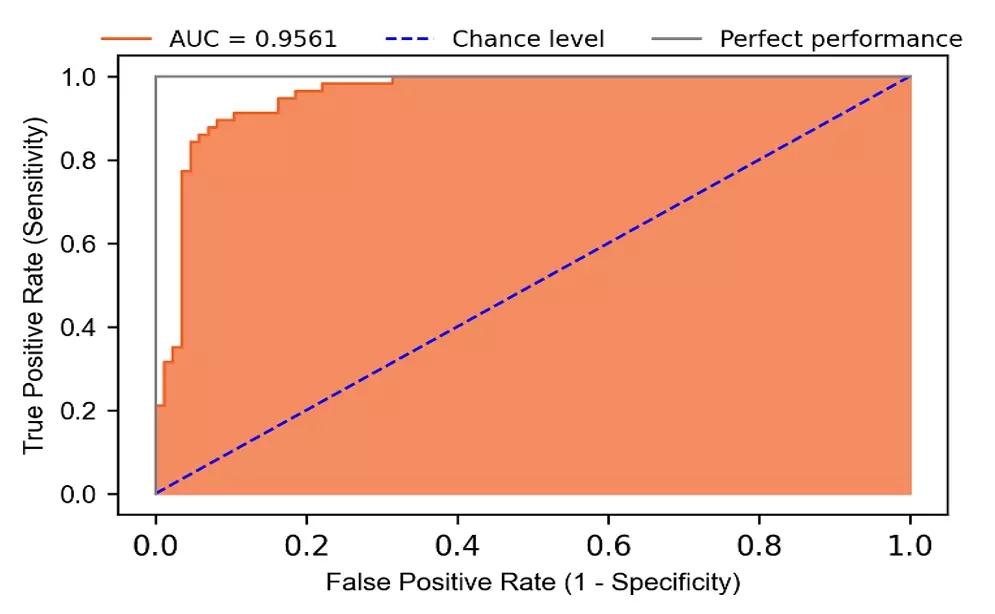## Logistic regression in Python (feature selection, model fitting, and prediction)

Logistic regression for prediction of breast cancer, assumptions, feature selection, model fitting, model accuracy, and interpretation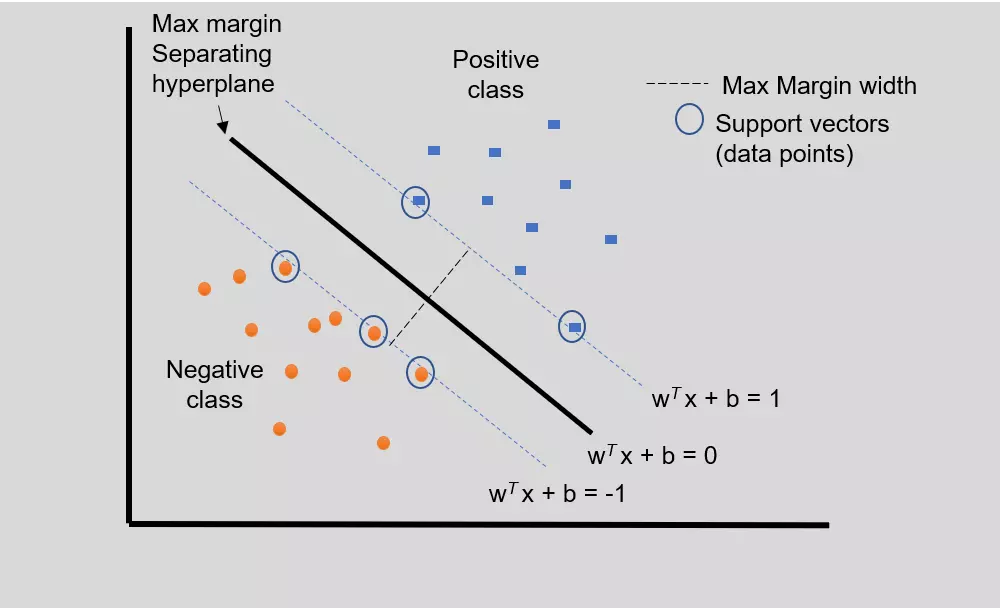## Support Vector Machine (SVM) basics and implementation in Python

Implementation of Support vector machine (SVM) in Python for prediction of heart disease. Learn SVM basics, model fitting, model accuracy, and interpretation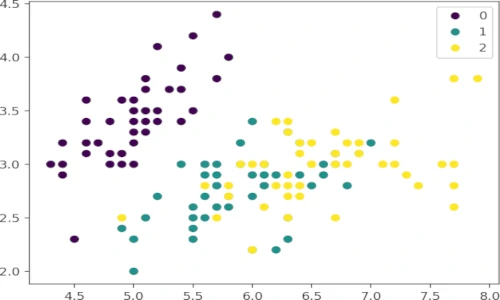## Create scatter plots using Python (matplotlib `pyplot.scatter`)

Learn how to create 2D and 3D scatter plots from numerical arrays and pandas DataFrame using Python matplotlib package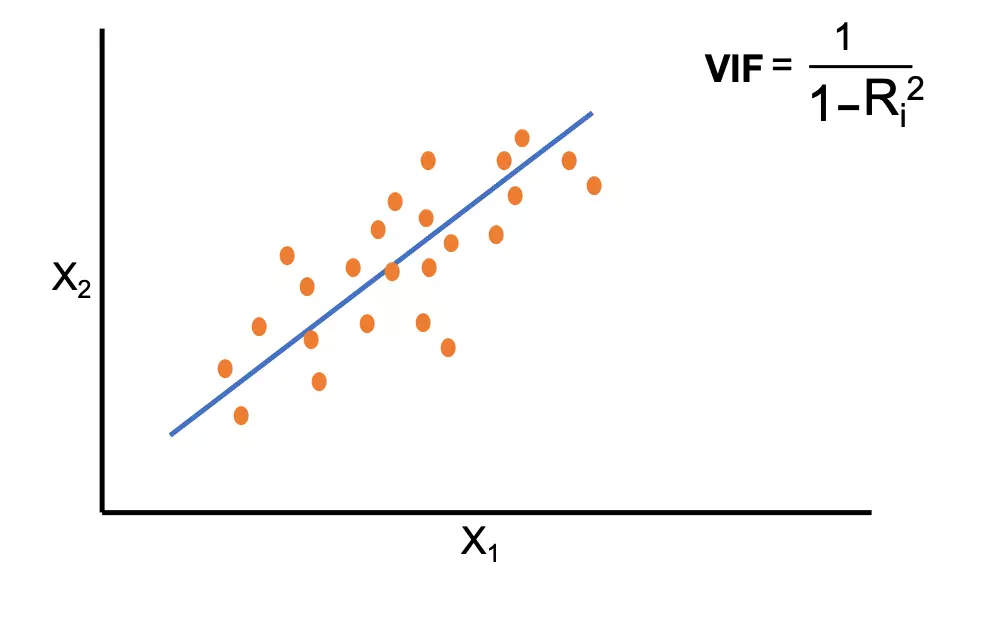## Multicollinearity and variance inflation factor (VIF) in the regression model (with Python code)